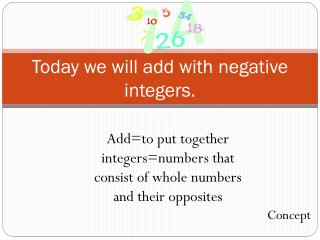DownloadDownload PresentationToday we will add with negative integers.

# Today we will add with negative integers.

Télécharger la présentation## Today we will add with negative integers.

- - - - - - - - - - - - - - - - - - - - - - - - - - - E N D - - - - - - - - - - - - - - - - - - - - - - - - - - -
##### Presentation Transcript

1. Today we will add with negative integers. Add=to put together integers=numbers that consist of whole numbers and their opposites Concept

2. When you get older and you start to “collect” credit cards and you start to buy things on “credit” you will be borrowing from the banks. • You buy an iPod for \$199.00 • You now owe \$199.00 (-199) • You buy a laptop for \$599.00 • You now owe \$599.00 (-599) • All together you owe \$798.00 • (-798) APK

3. Add • Add means to put together. \$199.00 + \$299.00=\$498.00 \$300.00 + \$600.00=\$900.00 Concept

4. Integer • numbers that consist of whole numbers and their opposites +5 -5 +500 -500 5 500 Concept

5. Absolute Value The absolute value of an integer is its distance from zero on the number line.For example, |-3| = 3 and |-5| = 5.

6. Importance • It will help you be a good “borrower” since you know how much you owe and how much you will have in order to pay. • It will be on tests and you need to know how to do it! • What would be other reasons to know how to add integers? IMP

7. What does this look like on a number line? +9 + -8 SD

8. Let’s try one! George owes his friend Jeanne \$3. If he borrows another \$6, how much will he owe her altogether? -3 + -6 -9 SD

9. +2 + - 7 -5 SD

10. +5 + - 7 1.  Find the absolute value of each integer. 2. Subtract the smaller number from the larger number you get in Step 1. 3. The result from Step 2 takes the sign of the integer with the greater absolute value. -2 SD

11. +9 + - 5 1.  Find the absolute value of each integer. 2. Subtract the smaller number from the larger number you get in Step 1. 3. The result from Step 2 takes the sign of the integer with the greater absolute value. +4 SD

12. -12 + + 5 1.  Find the absolute value of each integer. 2. Subtract the smaller number from the larger number you get in Step 1. 3. The result from Step 2 takes the sign of the integer with the greater absolute value. 12 & 5 12 – 5 7 -7 GP

13. -15 + + 15 1.  Find the absolute value of each integer. 2. Subtract the smaller number from the larger number you get in Step 1. 3. The result from Step 2 takes the sign of the integer with the greater absolute value. 15 & 15 15 – 15 0 0 GP

14. +11 + -13 1.  Find the absolute value of each integer. 2. Subtract the smaller number from the larger number you get in Step 1. 3. The result from Step 2 takes the sign of the integer with the greater absolute value. 11 & 13 13 – 11 2 -2 GP

15. Let’s review! • What does add mean? To put together. • What is an integer? numbers that consist of whole numbers and their opposites. What is the absolute value of -15? Of -6? Concept